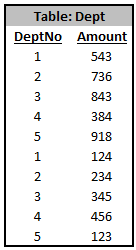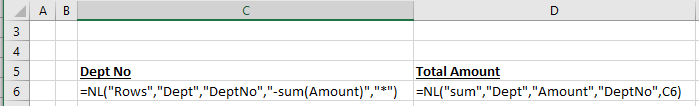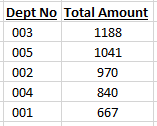Community
Training
Submit a Request
Become a Jet Insider
Give Feedback

# Sort by Sum for data sources other than NAV

When working with a data source other than Dynamics NAV (for example, a SQL database), sorting by the Sum of a field is quite simple.

Let's assume the following data is in the DEPT table of our database:and we want to list our department numbers, sorted by the decreasing value of the sum of the Amount field.

Our functions would look like this:with the results of: